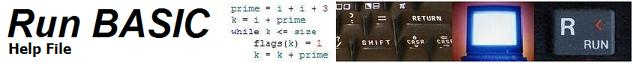FOR/NEXT and EXIT FOR

Description:

The FOR . . . NEXT looping construct provides a way to execute code a specific amount of times. See the section below on the proper way to exit a loop before the counter variable reaches the limit. A starting and ending value are specified:

for var = 1 to 10
{BASIC code}
next var

In this case, the {BASIC code} is executed 10 times, with var being 1 the first time, 2 the second, and on through 10 the tenth time. Optionally (and usually) var is used in some calculation(s) in the {BASIC code}. For example if the {BASIC code} is print var ^ 2, then a list of squares for var will be displayed upon execution.

The specified range could just as easily be 2 TO 20, instead of 1 TO 10, but since the loop always counts +1 at a time, the first number must be less than the second. The way around this limitation is to place STEP n at the end of for FOR statement:

for index = 20 to 2 step -1
{BASIC code}
next index

This loops 19 times returning values for index that start with 20 and end with 2. STEP can be used with both positive and and negative numbers and it is not limited to integer values. For example:

for x = 0 to 1 step .01
print "The sine of "; x; " is "; sin(x)
next x

Optional variable with NEXT

Run BASIC makes the use of a variable after "next" optional, but if one is designated, it must match the variable use with "for". Example:

Correct:

for x = 0 to 1 step .01
print "The sine of "; x; " is "; sin(x)
next

Incorrect:

for x = 0 to 1 step .01
print "The sine of "; x; " is "; sin(x)
next y

Exiting a loop prematurely

GOSUB and CALL may be used within a FOR/NEXT loop because they only temporarily redirect program flow or call on other parts of the program. Program execution resumes within the FOR/NEXT loop in these instances. Program execution does not return to the FOR/NEXT loop if GOTO is used within the loop.

While it is acceptable in Run BASIC to use GOTO to exit a FOR/NEXT loop, it is not possible to use GOTO to jump into a loop.

EXIT FOR

If it is necessary to exit a loop before the counter variable has reached its final value, use the EXIT FOR statement. This allows the program to exit the loop properly and to preserve the current value of the counter variable. Use it like this:

for x = 1 to 20
y=x*3
if y>40 then EXIT FOR
next x
print "Final x value ";x
print "Final y value ";y

Output:

Final x value 14

Final y value 42